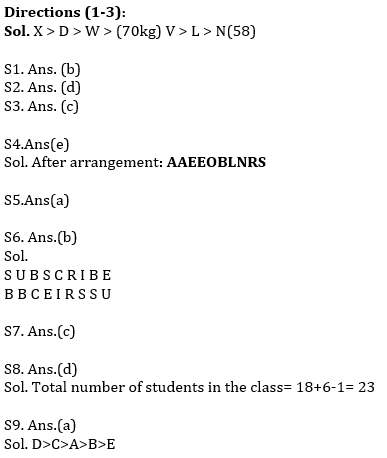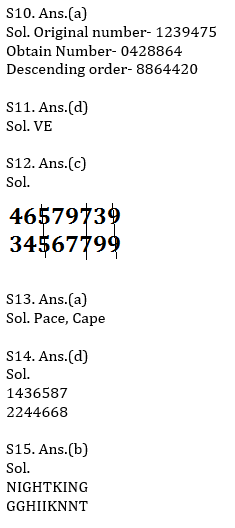Latest Banking jobs   »   Reasoning Ability Quiz For SBI/IBPS PO...

# Reasoning Ability Quiz For SBI/IBPS PO Prelims 2021- 15th October

Directions (1-3): Read the following information carefully and answer the given questions.
D, L, N, X, W and V are six friends. Each of them has different weight. L is heavier than only N. W is lighter than only two people. V is not the heaviest. The difference between the weight of third lightest and the lightest person is 12kg. D is heavier than W but is not the heaviest. The weight of V is 70 kg.

Q1. How many persons are heavier than V?
(a) Four
(b) Three
(c) Two
(d) One
(e) None of these

Q2. Which of the following statement is true if weight of L is 66 kg?
(a) The difference between the weight of V and L is 6 kg.
(b) Weight of W is 65 kg.
(c) Weight of D is 24 kg.
(d) The difference between the Weight of L and N is 8 kg.
(e) None is true

Q3. What could be the possible Weight of W?
(a) 62 kg
(b) 66 kg
(c) 80 kg
(d) 69 kg
(e) 59 kg

Q4. If all the letters in the word Reasonable are arranged in alphabetical order from left to right in such a way that all vowels are arranged first followed by consonants, then how many letters are there in between O and S after the arrangement?
(a) Two
(b) One
(c) None
(d) Three
(e) Four

Q5. If in the number 639429687, 1 is added to each of the digit which is less than five and 1 is subtracted from each of the digit which is greater than five then how many digits are repeating in the number thus formed?
(a) Two
(b) One
(c) None
(d) Three
(e) Four

Q6. The position of how many alphabets will remain unchanged if each of the alphabets in the word ‘SUBSCRIBE’ is arranged in alphabetical order from left to right?
(a) None
(b) One
(c) Two
(d) Three
(e) More than Three

Q7. What will come in place of question-mark (?) in the following series?
AZF BYG CXH DWI ?
(a) ESJ
(b) EUV
(c) EVJ
(d) DST
(e) FRH

Q8. Priya rank is 18th from the top and 6th from the bottom in the class. How many students are there in the class?
(a) 29
(b) 28
(c) 27
(d) 23
(e) None of these

Q9.Among A, B, C, D and E, A is heavier than B but lighter than C. B is heavier than only E. C is not the heaviest. Who among them will be in the middle if they stand in the order of their weight?
(a) A
(b) C
(c) B
(d) D
(e) E

Q10. If 2 is multiply by all the even digits and 1 is Subtracted from all the odd digits in the number 1239475 then arrange all digits in descending order, then which of the following is second from the right end?
(a) 2
(b) 8
(c) 6
(d) 4
(e) 0

Q11. What should come in place of question mark (?) in the following series based on the above arrangement?
ZA YB XC WD ?
(a) EU
(b) LO
(c) LP
(d) VE
(e) None of these

Q12. How many number will be remain at the same position in the given number “46579739” when they are arranged in the ascending order from left to right?
(a) One
(b) Two
(c) Three
(d) More than three
(e) None of these.

Q13. If it is possible to make only one meaningful word with the 1st ,2nd ,4th and 7th letters of the word ‘CAREERPOWER’ which would be the third letter of the word from the right? If more than one such word can be formed give ‘Y’ as the answer. If no such word can be formed, give ‘Z’ as your answer.
(a) Y
(b) P
(c) C
(d) Z
(e) M

Q14. If 1 is added to each odd digit and 2 is subtracted from each even digit in the number 1436587, then how many digits will appear twice in the new number thus formed?
(a) Only 8
(b) Only 8 and 6
(c) 8, 6 and 4
(d) 2, 4 and 6
(e) None of these

Q15. How many letters will be remaining at the same position in the word ‘NIGHTKING’ when letters arranged according to alphabetical order from left to right?
(a) One
(b) Two
(c) Three
(d) More than Three
(e) None

Solutions×

Thank You, Your details have been submitted we will get back to you.Join India's largest learning destination

What You Will get ?

•Job Alerts
•Daily Quizzes
•Subject-Wise Quizzes
•Current Affairs
•Previous year question papers
•Doubt Solving session

ORJoin India's largest learning destination

What You Will get ?

•Job Alerts
•Daily Quizzes
•Subject-Wise Quizzes
•Current Affairs
•Previous year question papers
•Doubt Solving session

ORJoin India's largest learning destination

What You Will get ?

•Job Alerts
•Daily Quizzes
•Subject-Wise Quizzes
•Current Affairs
•Previous year question papers
•Doubt Solving session

Enter the email address associated with your account, and we'll email you an OTP to verify it's you.Join India's largest learning destination

What You Will get ?

•Job Alerts
•Daily Quizzes
•Subject-Wise Quizzes
•Current Affairs
•Previous year question papers
•Doubt Solving session

Enter OTP

Please enter the OTP sent to
/6

Did not recive OTP?

Resend in 60sJoin India's largest learning destination

What You Will get ?

•Job Alerts
•Daily Quizzes
•Subject-Wise Quizzes
•Current Affairs
•Previous year question papers
•Doubt Solving sessionJoin India's largest learning destination

What You Will get ?

•Job Alerts
•Daily Quizzes
•Subject-Wise Quizzes
•Current Affairs
•Previous year question papers
•Doubt Solving session

Almost there

+91Join India's largest learning destination

What You Will get ?

•Job Alerts
•Daily Quizzes
•Subject-Wise Quizzes
•Current Affairs
•Previous year question papers
•Doubt Solving session

Enter OTP

Please enter the OTP sent to Edit Number

Did not recive OTP?

Resend 60

By skipping this step you will not recieve any free content avalaible on adda247, also you will miss onto notification and job alerts

Are you sure you want to skip this step?

By skipping this step you will not recieve any free content avalaible on adda247, also you will miss onto notification and job alerts

Are you sure you want to skip this step?